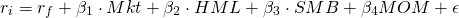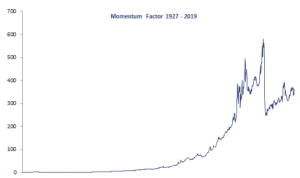# Carhart 4 Factor model

The Carhart 4 Factor model is a popular multifactor model used to price securities. the Carhart model is an extension of the Fama and French 3-factor model. It was proposed by Mark Carhart in 1997. The Carhart four-factor model includes a cross-sectional momentum factor that improves the explanatory power of the multifactor model considerably.

On this page, we discuss the Carhart factor model, provide the Carhart 4-factor model equation, and illustrate how to use the Carhart momentum model. At the bottom of the page, a Carhart four factor model Excel spreadsheet is available for download. In the spreadsheet, we use data provided by Kenneth French.

## Carhart 4 factor model (1997)

The Carhart 4 factor model was proposed a few years after the Fama-French 3 factor model was introduced (1993). The factor, cross-sectional momentum, was not discovered by Carhart, but by Jegadeesh and Titman a few years earlier. Carhart nevertheless showed that the momentum factor was distinct from the Fama and French (1993) factors and that it improved the explanatory of multifactor models aimed at explaining mutual funds’ performance. Since the model augments the Fama-French model, a better name of the model would be the Fama French Carhart factor model.

## Carhart 4 factor model equation

Next, let’s have a look at the equation. The full Carhart model looks as followswhere Mkt is the return on the market portfolio, HML is the book-to-market factor, SMB is the size factor, and MOM is the momentum factor. Sometimes, researchers refer to the latter factor as UMD, which stands for Up-minus-Down. The Carhart model can easily be estimated using OLS. The only thing we require is time-series for the different factors. While calculating these factors ourselves is cumbersome, they are made available for download by researchers.

## Carhart four factor model

Finally, let’s have a look at the Carhart four factor model Excel implementation. The following figure plots the returns of the cross-sectional momentum factor for the US. Interestingly, we notice that the momentum factor crashed on two occasions. While the first case is hard to distinguish, the second crash in 2008 is clearly visible. These are referred to as momentum crashes. This is a phenomenon that was first described by Kent Daniel in 2011.## Summary

We discussed the Carhart 4 factor model. The model is an extension of the Fama-French model that considerably improves the fit of multifactor models in pricing securities.

### Return calculation

Want to have an implementation in Excel? Download the Excel file: Carhart 4 factor data